﻿ 低速AUV航渡过程中减少洋流影响的方法
 舰船科学技术2020, Vol. 42Issue (2): 88-92, 97PDF

1. 中国人民解放军92493部队，辽宁 葫芦岛 125000;
2. 中船重工七〇五研究所昆明分部，云南 昆明 650102

Adaptive decision methods to reduce effect on ocean current to low-speed AUV sailing
LIU Jia1, ZHOU Wei-jiang1, MA Wei-jian2
1. No. 92493 Unit of PLA, Huludao125000, China;
2. The 705 Research Institute Kunming Branch, Kunming 605118, China
Abstract: As a tool for human exploration of the ocean and the development of the ocean, Autonomous Underwater Vehicle (AUV) is widely used in marine scientific investigations, underwater surveys and other civil missions, as well as military tasks such as surveillance, investigation, and patrol. AUVs often use low-speed navigation, resulting in a greater impact on the marine environment, especially ocean currents. In this paper, the influence mechanism of ocean current on low-speed AUV is analyzed, and several methods to reduce the influence of ocean current are provided and analyzed. It is verified that the method of increasing the route control point can effectively reduce the influence of ocean current.
Key words: low-speed AUV     ocean current     sea route     speed-control     sailing direction control
0 引　言

1 洋流对低速AUV的影响 1.1 低速AUV的特点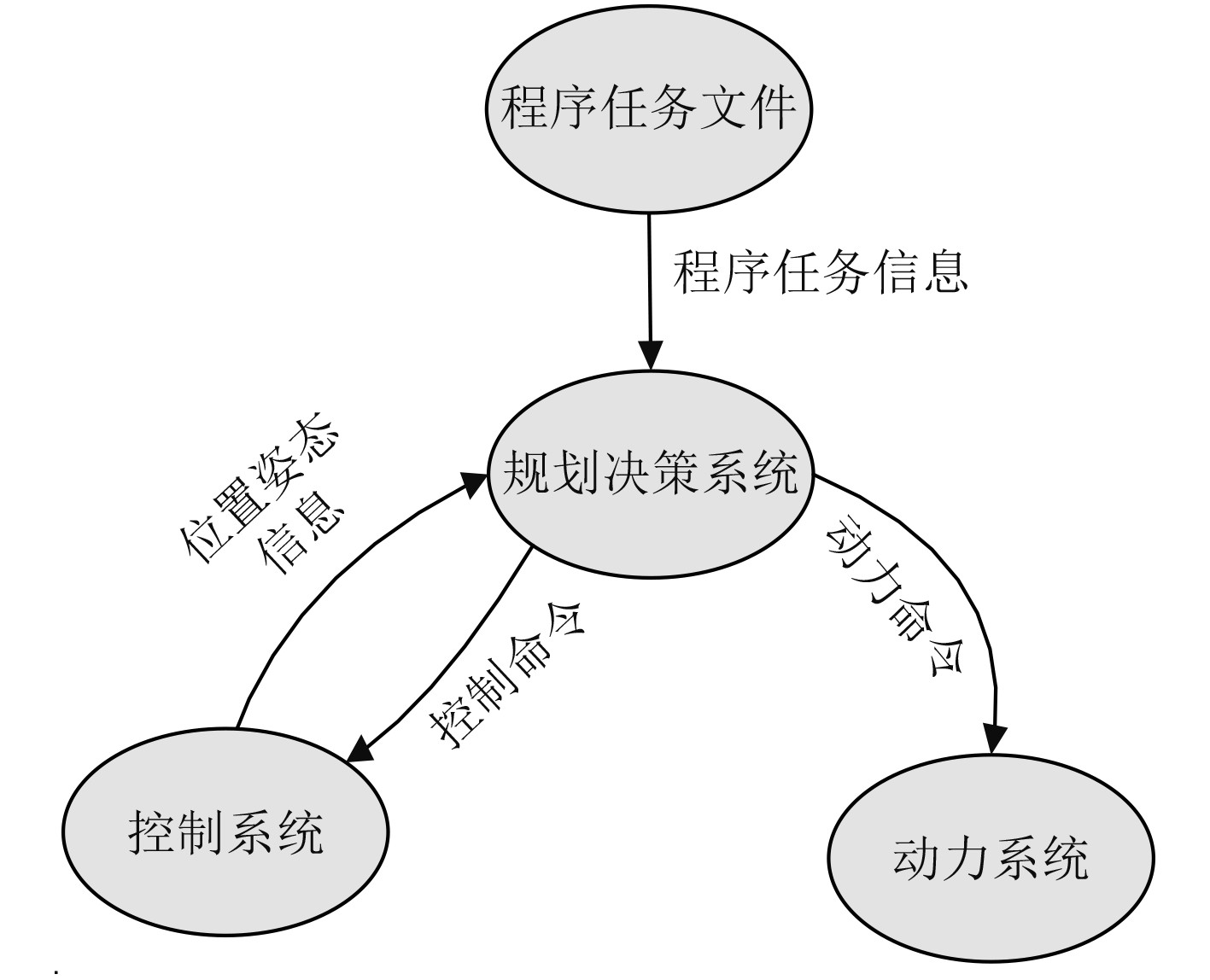图 1 AUV信息流向图 Fig. 1 Information flow direction figure of AUV
1.2 洋流对低速AUV航行的影响

1.3 实航影响分析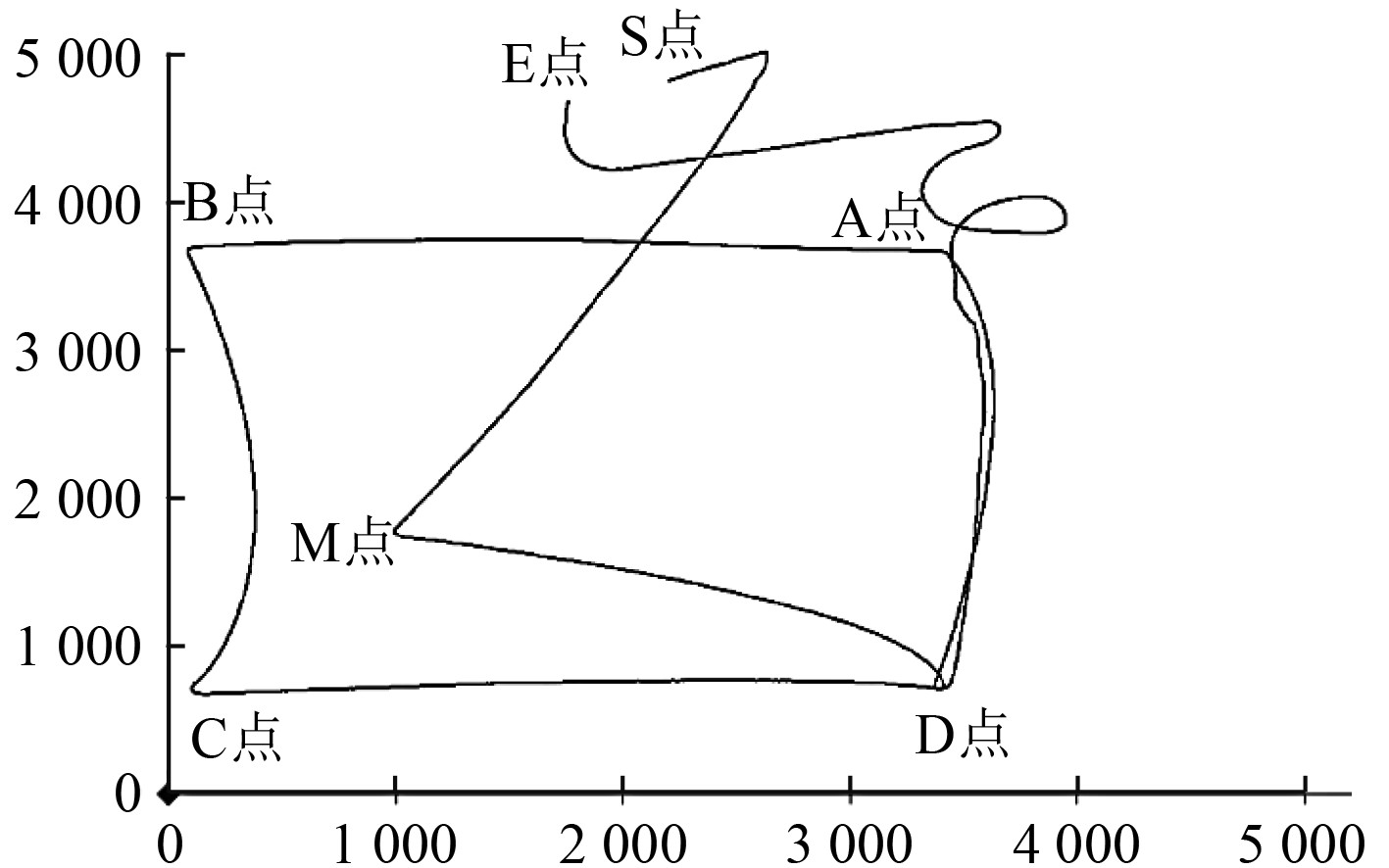图 2 低速AUV航行轨迹图 Fig. 2 Low-speed sailing tracks figure of AUV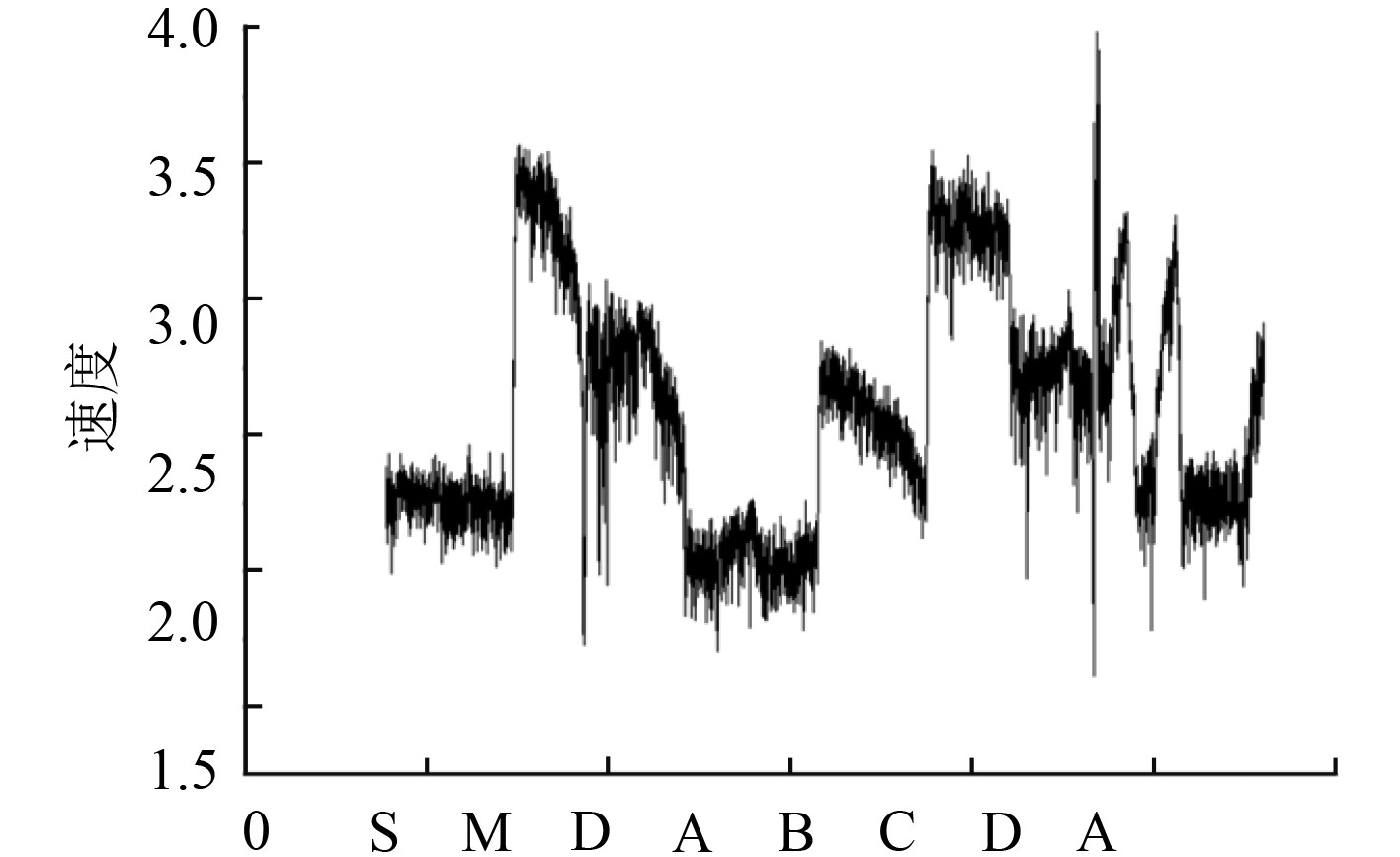图 3 AUV航速变化图 Fig. 3 Sailing speed change figure of AUV

2 潮流的特点及对低速AUV的影响机理分析 2.1 潮流的特点

2.2 潮流的运动及简化

 \left\{ \begin{aligned} & \frac{{\partial \delta }}{{\partial t}} + \frac{{\partial (hu)}}{{\partial x}} + \frac{{\partial (hv)}}{{\partial x}} = 0 {\text{，}} \\ & \frac{{\partial u}}{{\partial t}} - 2\omega \sin \lambda \cdot v = - \frac{\partial }{{\partial x}}(\varOmega + g\delta ) {\text{，}} \\ & \frac{{\partial v}}{{\partial t}} + 2\omega \sin \lambda \cdot u = - \frac{\partial }{{\partial y}}(\varOmega + g\delta ) {\text{。}} \end{aligned} \right. (1)

 \left\{ \begin{aligned} & \frac{{\partial u}}{{\partial t}} + g\frac{{\partial \delta }}{{\partial x}} = 0 {\text{，}} \\ & \frac{{\partial \delta }}{{\partial t}} + h\frac{{\partial u}}{{\partial x}} = 0 {\text{。}} \end{aligned} \right. (2)

 $\delta = a\sin (\omega t - kx) {\text{。}}$ (3)

 $u = C\frac{a}{h}\sin (\omega t - kx) = \frac{C}{h}\delta = \sqrt g \frac{\delta }{{\sqrt h }} {\text{。}}$ (4)

2.3 潮流对低速AUV的影响机理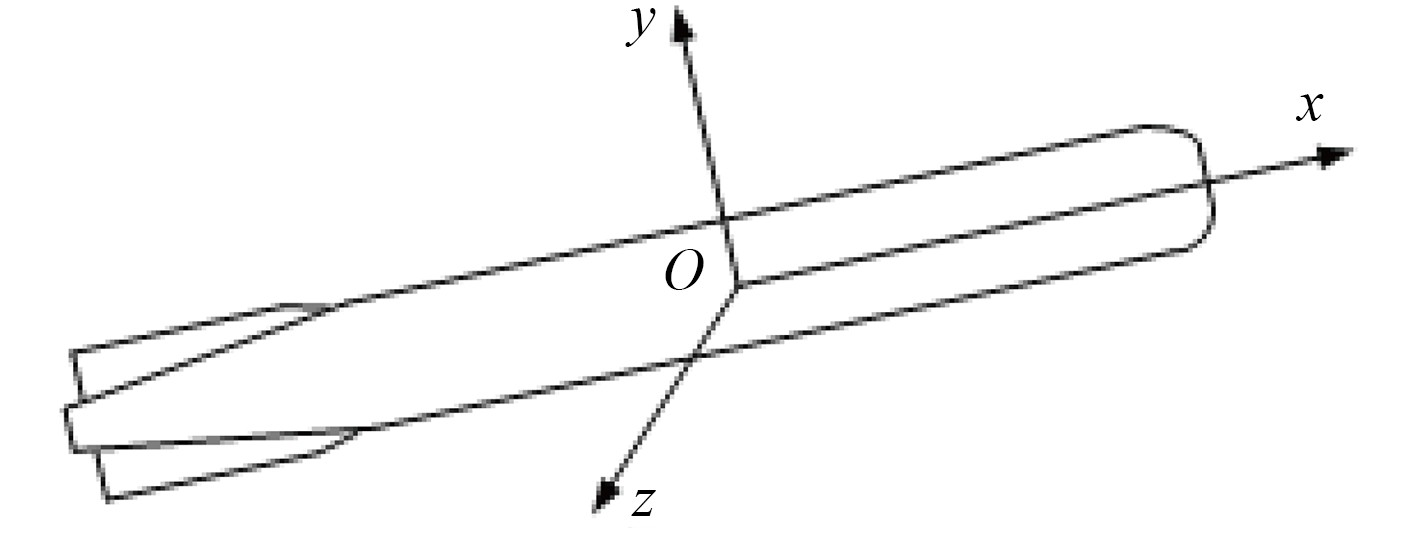图 4 低速AUV坐标分解图 Fig. 4 Coordinate decomposition figure of 低速AUV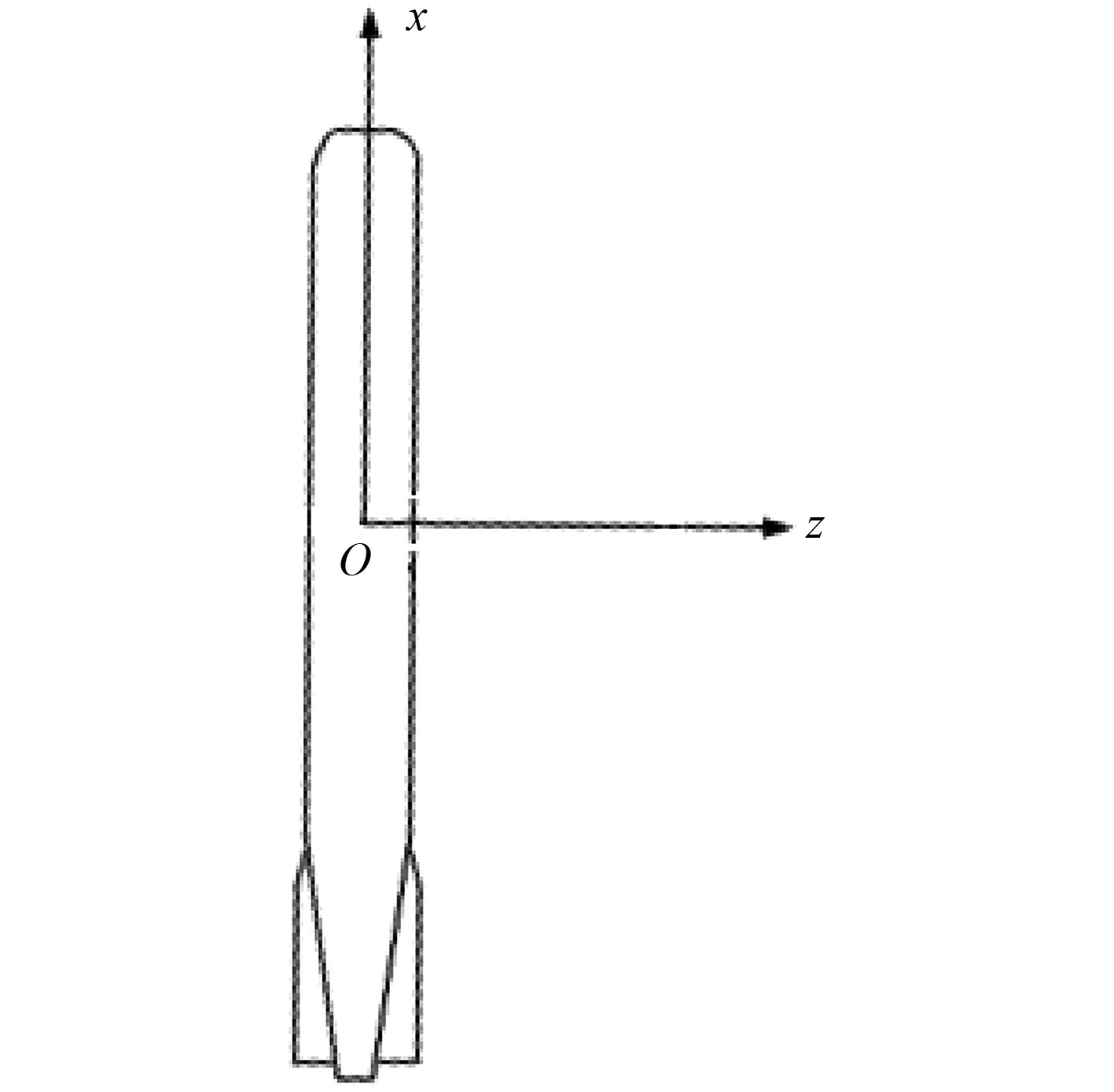图 5 受洋流影响示意图 Fig. 5 Effects on ocean current schematic diagram

 ${v_x} = {v_0} + {v_1} \cdot \cos (\theta - \varphi ) {\text{，}}$ (5)

 ${v_z} = {v_1} \cdot \sin (\theta - \varphi ) {\text{，}}$ (6)

 $\eta = \theta + {{{v_z}} / {{v_x}}} {\text{，}}$ (7)

 $v = \sqrt {{v_x}^2 + {v_z}^2} {\text{。}}$ (8)

3 减小潮流对低速AUV航渡影响探讨

1）减小洋流对低速AUV航速的影响。可采用速度闭环控制的方式来实现，即动力系统的控制目标不再是螺旋桨转速，而是低速AUV的航行速度。

2）减小洋流对低速AUV航迹偏移量的影响。从式（8）中分析应该是使 ${v_z} = 0$ ，这样低速AUV的航迹向等于航向，就不会偏离预设航路。由于实际航渡的需要，低速AUV不可能总是顺流或逆流航向，不可避免地会出现低速AUV流向和航向的差不等于0的情况，这就要求，低速AUV要在垂直航路方向产生速度分量，抵消洋流影响，使垂直航路方向合速度为0。

3.1 加装水平推进器

3.2 航路纠正

3.3 增加航路控制点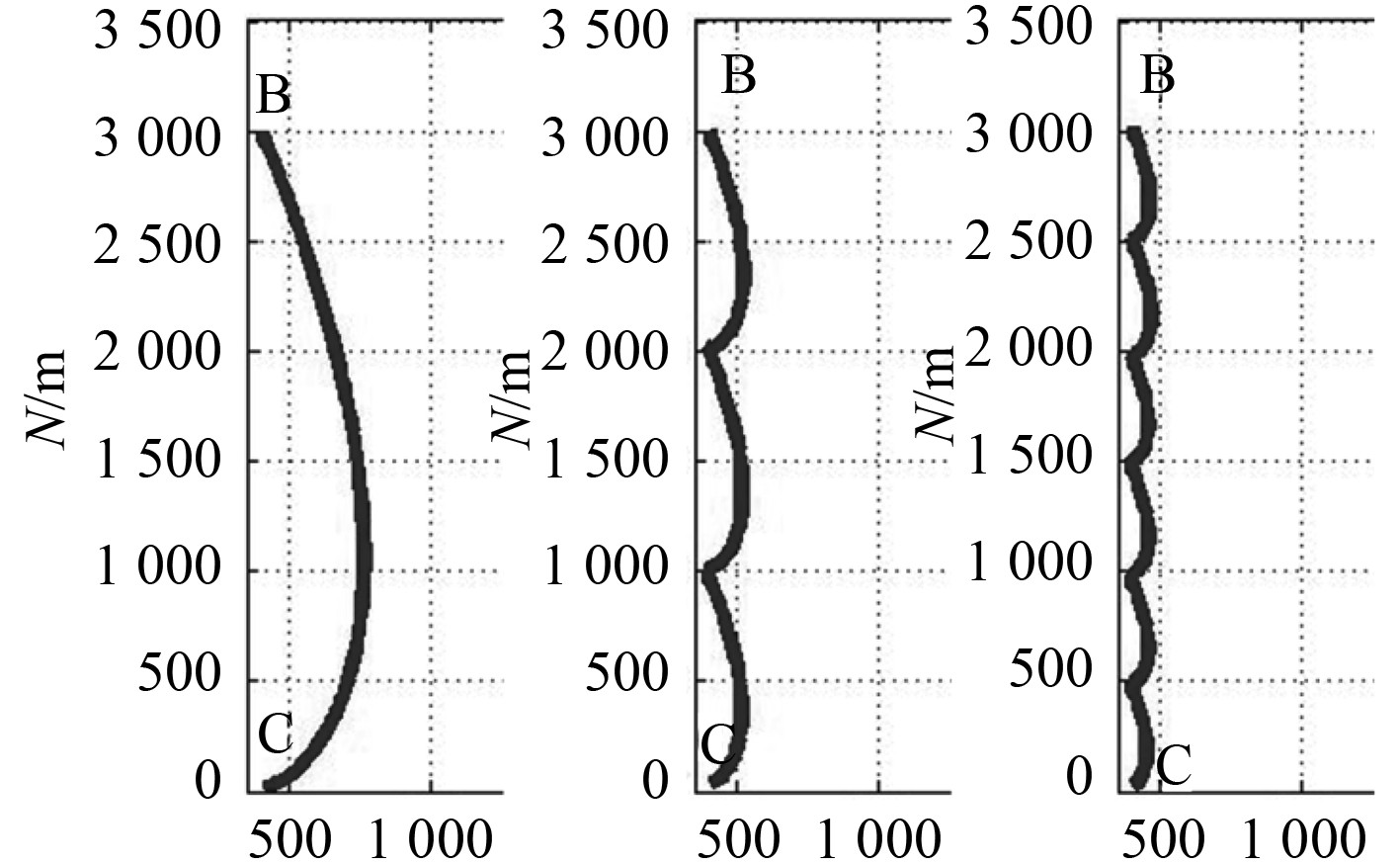图 6 不同航路控制点下的航迹图 Fig. 6 Tracks figure of different sailing control points

1）不插入航路控制点时，此时只有一个航路控制点，即点C，低速AUV到达目标点的时间较长，洋流对低速AUV影响效果较明显，航迹最大偏差约为750 m。

2）在中间每1 km处插入2个航路控制点时，此时有3个航路控制点（包括点C），洋流对低速AUV影响效果就减弱，航迹最大偏差约为500 m。

3）在中间每500 m处插入5个航路控制点时，此时共有6个航路控制点（包括点C），洋流对低速AUV影响效果就明显减弱，约为50 m，航迹最大偏差约为350 m。表 1 仿真实验不同航路控制点下的偏差统计 Tab.1 Deviation statistics of simulative experiment on different sailing control points condition
4 验　证表 2 实航实验条件 Tab.2 Real experiment condition

AUV从原点出发沿正西方向航行约22 km，每隔1 km插入一个航程控制点。实航实验的轨迹如图7所示。图8为实航实验的0～4.5 km部分轨迹图。由于实航实验成本较高，未进行实航对比实验，因此采用仿真的办法进行验证。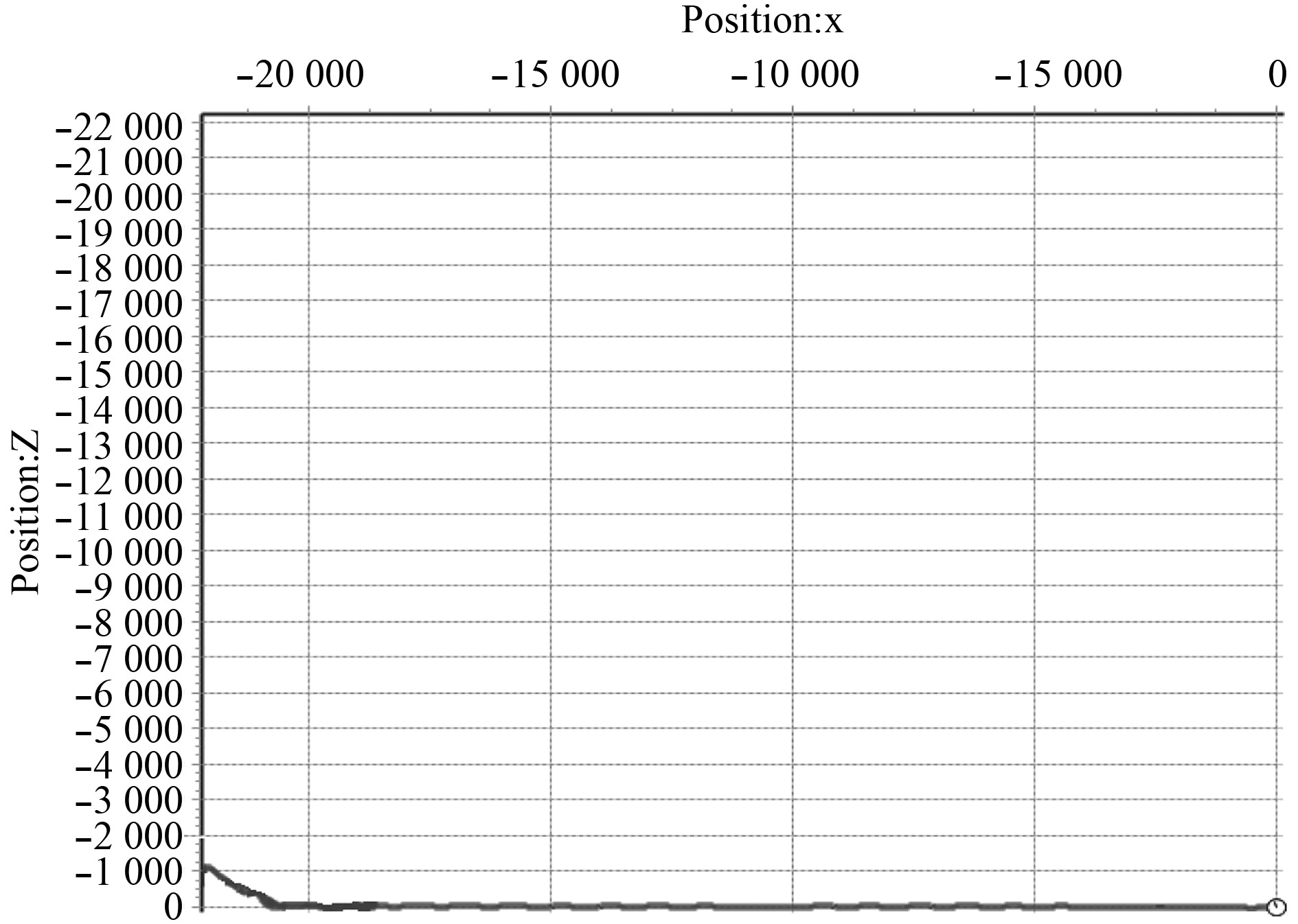图 7 AUV实航轨迹图 Fig. 7 Real experiment tracks figure of AUV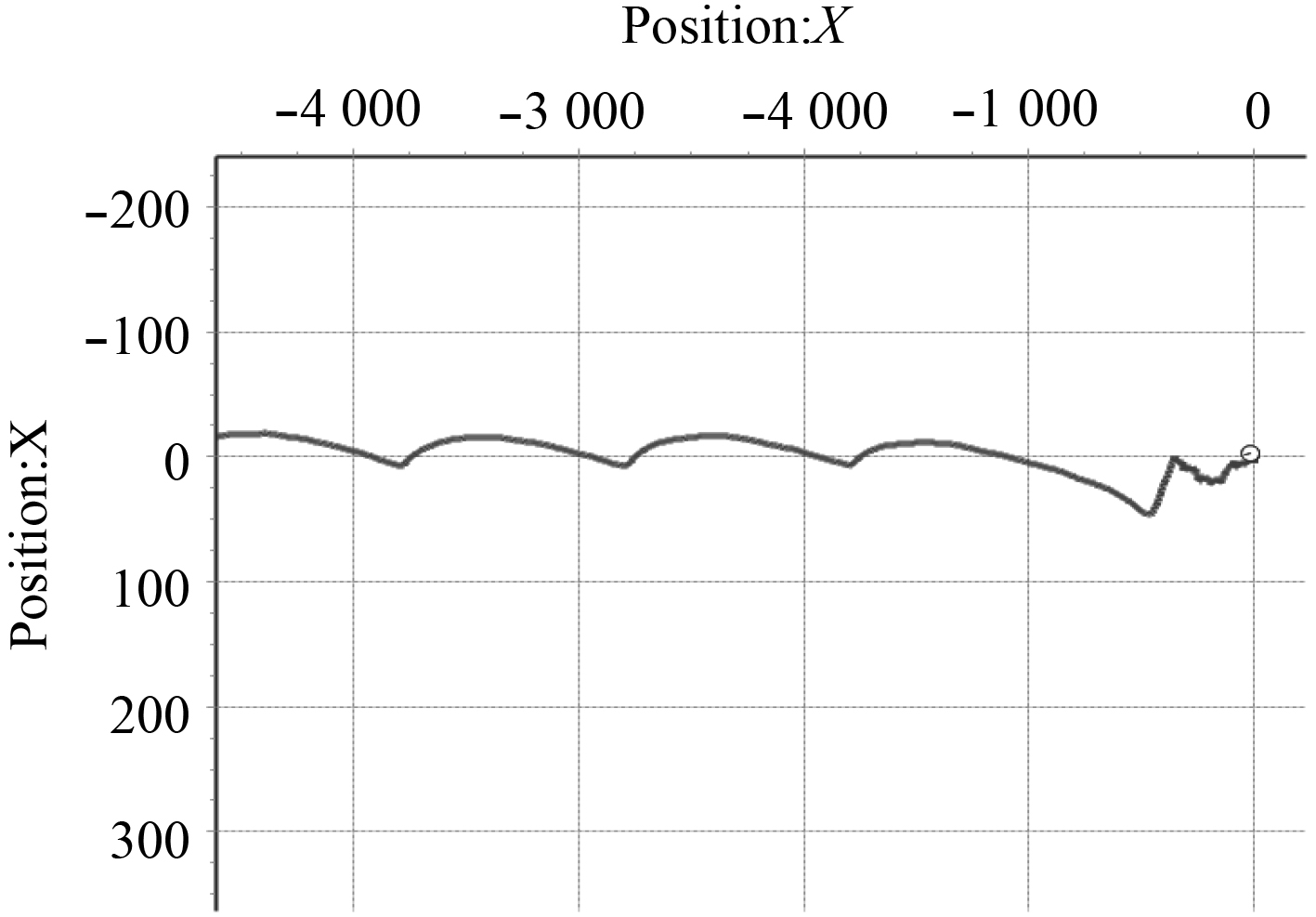图 8 AUV实航实验的0～4.5 km部分轨迹图 Fig. 8 0～4 km part of real experiment tracks figure of AUV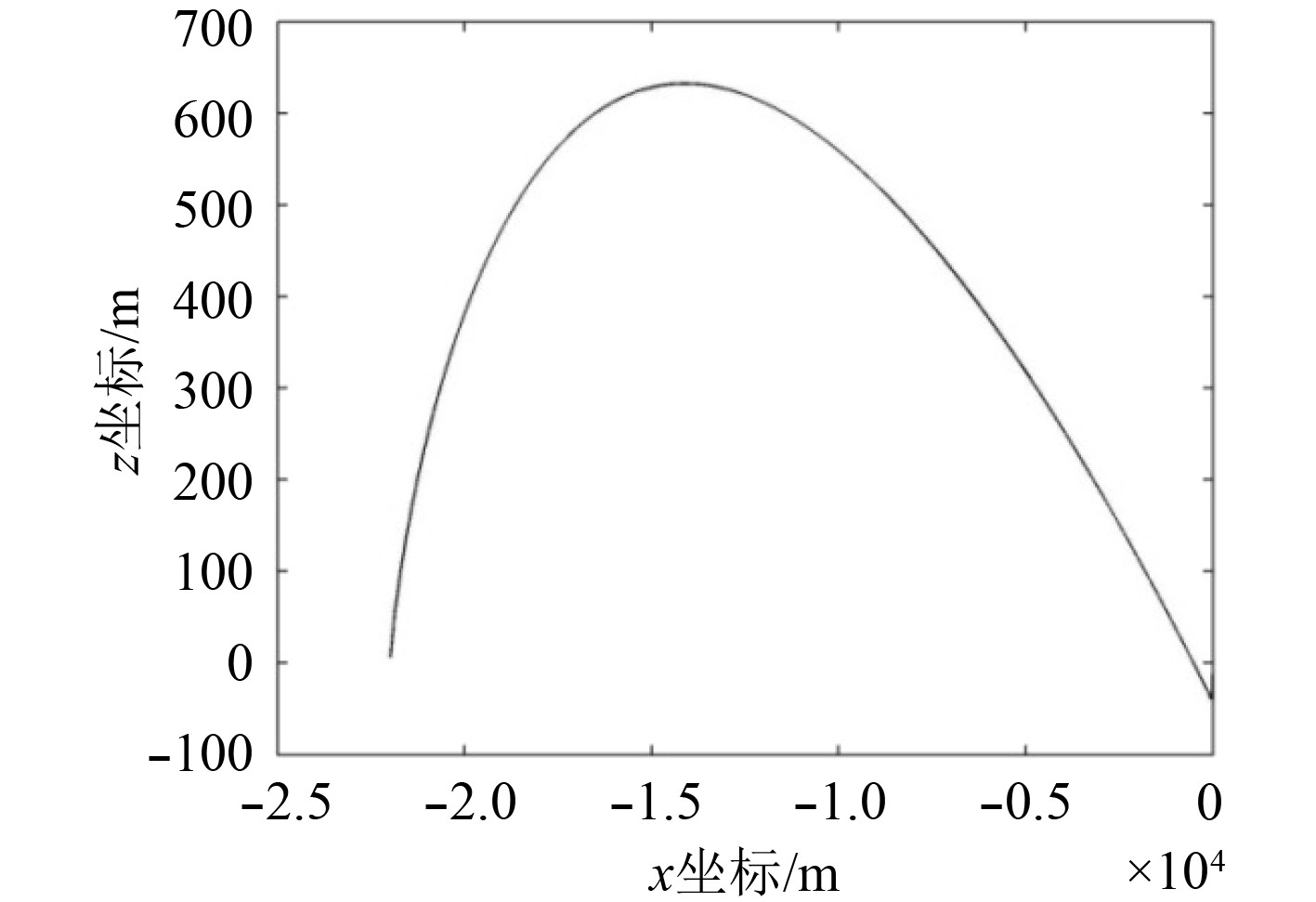图 9 AUV仿真实验轨迹图 Fig. 9 Simulative experiment tracks figure of AUV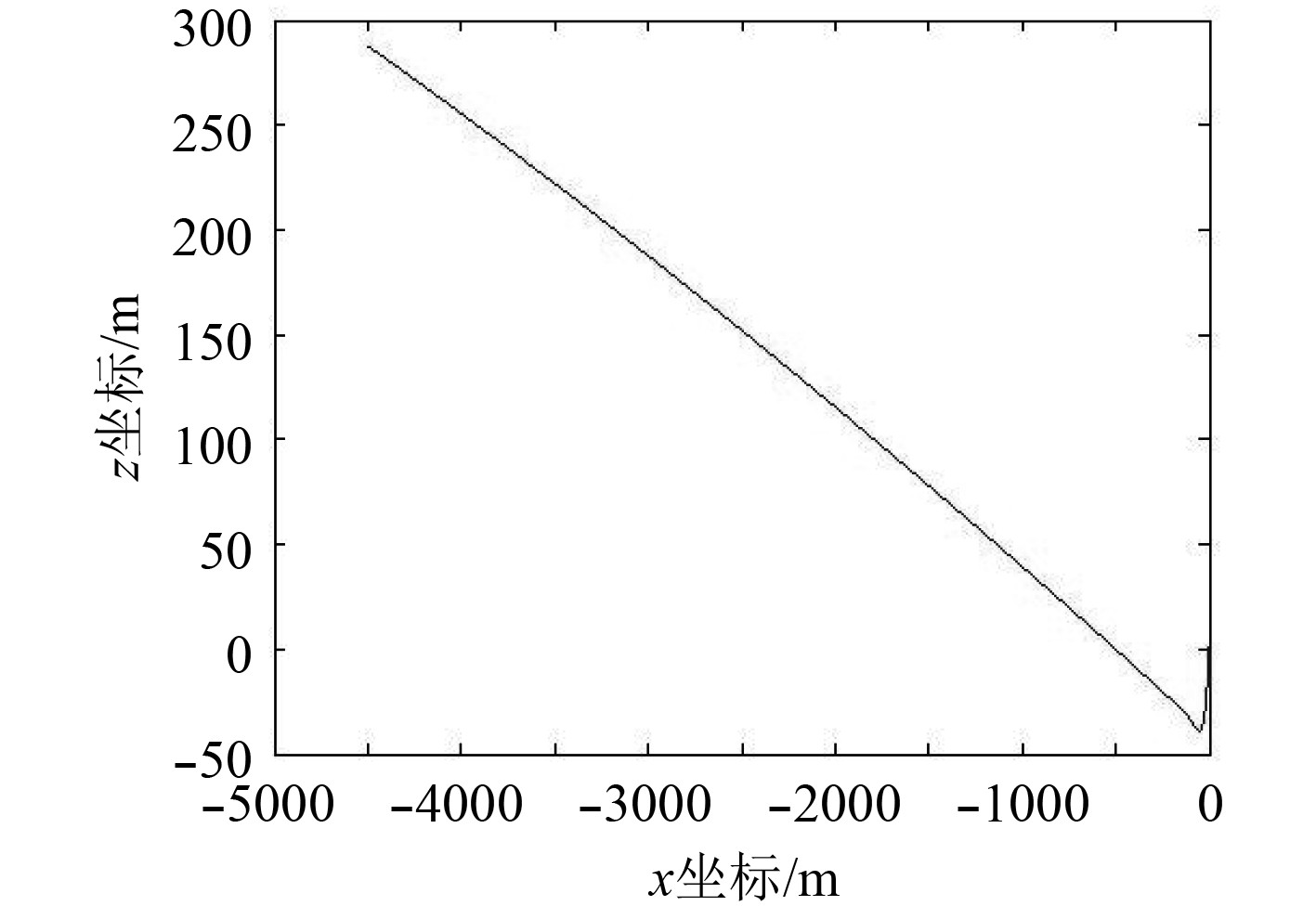图 10 AUV仿真实验0～4.5 km部分轨迹图 Fig. 10 Simulative experiment 0～4 km tracks figure of AUV表 3 实航实验与仿真的对比 Tab.3 Comparation between real experiment result and simulative experiment result
5 结　语

本文通过分析AUV在航行阶段受到洋流影响现象，探究了洋流对AUV的影响机理，有针对性地提出几种减小洋流影响的方案，并对预设航路增加控制点的方案进行仿真验证。仿真结果证明预设航路控制点的方法能有效减小洋流对低速AUV航迹偏移量的影响，降低航迹偏差率，使航迹更接近于直线。该方法在实航试验中也得到了验证，并已得到实际工程应用。

  高富东. 复杂海况下新型AUV设计与关键技术研究[D]. 长沙: 国防科技大学, 2012  范双双. 洋流影响下的水下滑翔机动力学建模、运动分析与控制器研究[D]. 杭州: 浙江大学, 2013.  房萍萍. 计及侧向流影响的自治AUV6_DOF运动仿真[D]. 天津: 天津大学, 2014.  陈玉. 复杂海洋环境下行形体的水动力特性研究[D]. 哈尔滨: 哈尔滨工程大学硕, 2012  姚鹏, 解则晓. 基于修正导航向量场的AUV自主避障方法[J/OL]. 自动化学报, 1-11[2019-03-19]. https://doi-org-443.e.buaa.edu.cn/10.16383/j.aas.c180219  苗润龙, 庞硕, 姜大鹏, 等. 海洋自主航行器多海湾区域完全遍历路径规划[J]. 测绘学报, 2019(2): 256-264.  鲁燕. 海流环境中水下机器人实时运动规划方法研究[D]. 哈尔滨: 哈尔滨工程大学, 2006.  YOERGER D, SLOTINE J. Robust trajectory control of underwater vehicles[J]. IEEE J ocean eng, 1985, 10(4): 462-470. DOI:10.1109/JOE.1985.1145131  LEVANT, ARIE. Sliding order and sliding accuracy in sliding mode control. International journal of control[J]. 1993, 58(6): 1247-1263.  LEVANT, ARIE. Higher-orderslidingmodes, differentiationandoutput-feedbackcontrol[J]. International journal of control, 2003, 76(9-10): 924-941. DOI:10.1080/0020717031000099029  SALGADO-JIMENEZ T, SPIEWAK J M, FRAISSE P, et al. Arobust control algorithm for AUV: based on a high order sliding mode[J]. IEEEtechno-ocean’04, 2004, 276-281.  KHAN I, BHATTI AI, KHAN Q, et al. Sliding mode control of lateral dynamics of an AUV[C]// Proceedings of 2012 9th International Bhurban Conference on Applied Sciences Technology, 2012: 27-31.